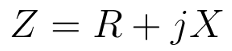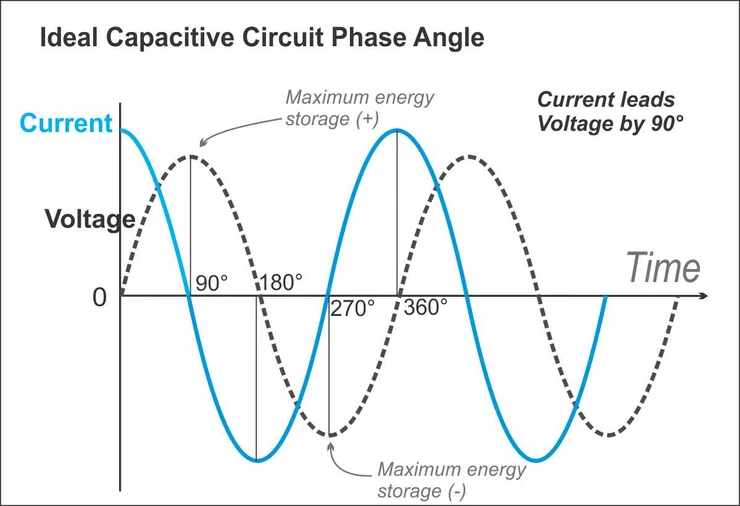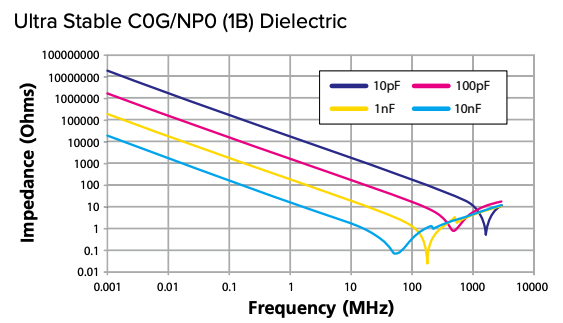Impedance, measured in ohms, extends the concept of “opposition” to alternating current (AC) applications. It accounts for resistance, the opposition of current flow, and reactance, the measure of opposing alternating current – an effect of inductance and/or capacitance. In direct current (DC) applications, we talk in terms of resistance, not reactance. Chances are: This isn’t new information. But there’s a reason we wanted to cover this topic – impedance values play an important role in capacitor selection.

Impedance (Z) can be expressed in a number of different ways. In Figure 1, impedance is shown as a complex number, where “R” represents resistance, “X” is reactance, and “j” is the imaginary number √(-1):Figure 1. Formula for calculating impedance in any circuit.

#### Connecting Capacitance and Impedance

Unlike reactance (X), which flows in two directions to represent AC current flow, resistance (R) flows in a single direction. Resistors in a circuit (implementing resistance) have voltage drops across them and dissipate power in the form of heat, while reactance stores energy as an electric field. Capacitors store that electrical energy for a temporary period. In time domain, voltage lags behind current by 90 degrees. So, in a circuit that includes a voltage source and a capacitor, maximum current flow occurs right when the circuit is connected because, at this point, the capacitor is empty. Current flow approaches 0 amperes as the capacitor gets closer and closer to its maximum capacity. At maximum capacity, the voltage of the capacitor is equal to the voltage source, and the capacitor starts to oppose current flow because current can’t continue to flow by. Figure 2 demonstrates the 90-degree phase shift between voltage and current.Figure 2.Voltage and current vs. time for a capacitor. Source.

Impedance is critical to understanding current opposition at specific operating frequencies. As mentioned, impedance accounts for resistance and reactance – AC and DC – which is particularly important for those working with AC circuits. In AC circuits, capacitors can become controllers and regulators of electrical flow. Component selection often depends on a specified frequency, so it’s important to know these specifications from the start. As shown in Figure 3, impedance values also indicate where signals will be blocked (or not) in signal blocking and bypass applications.Figure 3. At the Self-Resonant Frequency, where the graph dips, the impedance is the equivalent series resistance (ESR) value.

Topics: Capacitor

## Insights Into Precision Passive Devices

Knowles Precision Devices is a premier global source for Capacitors, RF Filters, EMI Filters, Resonators, non-magnetic components and advanced dielectric materials. An umbrella for the brands of Compex, DLI, Johanson MFG, Novacap, Syfer and Voltronics, Knowles Precision Devices serves a variety of markets including military, aerospace/avionics, medical equipment, implantable devices, EMI and connector filtering, oil exploration, instrumentation, industrial electronics, automotive, telecoms and data networks.

This Blog will provide insights into:

• Filters for 5G and mmWave
• Capacitors for High Voltage
• Capacitors for High Reliability

and much more. Subscribe below to stay informed.